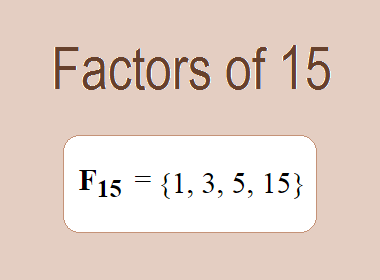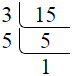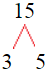# Factors of 15The factors of 15 are 1, 3, 5, and 15 are the factors of 15 i.e. F15 = {1, 3, 5, 15}. The factors of 15 are all the numbers that can divide 15 without leaving a remainder.

We can check if these numbers are factors of 15 by dividing 15 by each of them. If the result is a whole number, then the number is a factor of 15. Let's do this for each of the numbers listed above:

·        1 is a factor of 15 because 15 divided by 1 is 15.

·        3 is a factor of 15 because 15 divided by 3 is 5.

·        5 is a factor of 15 because 15 divided by 5 is 3.

·        15 is a factor of 15 because 15 divided by 15 is 1.

## How to Find Factors of 15?

1 and the number itself are the factors of every number. So, 1 and 15 are two factors of 15. To find the other factors of 15, we can start by dividing 15 by the numbers between 1 and 15. If we divide 15 by 2, we get a remainder of 1. Therefore, 2 is not a factor of 15. If we divide 15 by 3, we get a remainder of 0. Therefore, 3 is a factor of 15.

Next, we can check if 4 is a factor of 15. If we divide 15 by 4, we get a remainder of 3. Therefore, 4 is not a factor of 15. We can continue this process for all the possible factors of 15.

Through this process, we can find that the factors of 15 are 1, 3, 5, and 15. These are the only numbers that can divide 15 without leaving a remainder.

********************

********************

## Properties of the Factors of 15

The factors of 15 have some interesting properties. One of the properties is that the sum of the factors of 15 is equal to 24. We can see this by adding all the factors of 15 together:

1 + 3 + 5 + 15 = 24

Another property of the factors of 15 is that they are all odd numbers. This is because 15 is an odd number, and any even number cannot divide an odd number without leaving a remainder.

Another property of the factors of 15 is that the prime factor of 15 are 3 and 5 only.

## Applications of the Factors of 15

The factors of 15 have several applications in mathematics. One of the applications is in finding the highest common factor (HCF) of two or more numbers. The HCF is the largest factor that two or more numbers have in common. For example, to find the HCF of 15 and 20, we need to find the factors of both numbers and identify the largest factor they have in common. The factors of 15 are 1, 3, 5, and 15. The factors of 20 are 1, 2, 4, 5, 10, and 20. The largest factor that they have in common is 5. Therefore, the HCF of 15 and 20 is 5.

Another application of the factors of 15 is in prime factorization. Prime factorization is the process of expressing a number as the product of its prime factors. The prime factors of 15 are 3 and 5 since these are the only prime numbers that can divide 15 without leaving a remainder. Therefore, we can express 15 as:

15 = 3 × 5

We can do prime factorization by division and factor tree method also. Here is the prime factorization of 15 by division method,15 = 3 × 5

Here is the prime factorization of 15 by the factor tree method,15 = 3 × 5

## Conclusion

The factors of 15 are the numbers that can divide 15 without leaving a remainder. The factors of 15 are 1, 3, 5, and 15. The factors of 15 have some interesting properties, such as being odd numbers and having a sum of 24. The factors of 15 have several applications in mathematics, such as finding the highest common factor and prime factorization.